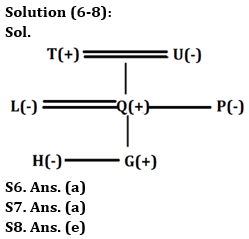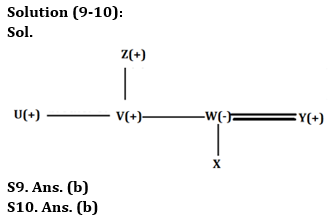Latest Banking jobs   »

# Reasoning Ability Quiz For Bank Foundation 2023 -27th April

Directions (1-5): Read the following arrangement of numbers, alphabets and symbols carefully and answer the questions based on it.

9 O 6 P & A 4 U S M # O 2 9 S A ? & # 2 Q 0 8 2 P @ 4 5 ! A 8 8 A \$

Q1. How many vowels are immediately followed by a number in the given arrangement?
(a) One
(b) Two
(c) Three
(d) Four
(e) More than four

Q2. If all the symbols are dropped then which element will be 6th from the left end after the rearrangement?
(a) U
(b) M
(c) 4
(d) A
(e) None of these

Q3. If all the numbers are dropped then which element will be 5th from the right end after the rearrangement?
(a) @
(b) Q
(c) P
(d) !
(e) None of these

Q4. What is the sum of 5th number from the left end and 3rd number from the right end in the given arrangement?
(a) 13
(b) 7
(c) 11
(d) 14
(e) None of these

Q5. How many numbers are immediately followed by vowel and immediately preceded by letter in the given arrangement?
(a) One
(b) Two
(c) Three
(d) Four
(e) More than four

Directions (6-8): answer the questions based on the information given below.
In a family, L is married to Q. U is the mother-in-law of L. U has two children and one of them is unmarried. P is the paternal aunt of G. T is the paternal grandfather of G who is the only son of L. H is child of Q. Q is a male.

Q6. How is H related to the daughter of U?
(a) Niece
(b) Nephew
(c) Either Niece or Nephew
(d) Son
(e) Daughter

Q7. Who is sister-in-law of P?
(a) L
(b) Q
(c) U
(d) T
(e) None of these

Q8. How many female members in the family?
(a) One
(b) Two
(c) Three
(d) Either two or three
(e) None of these

Directions (9-10): answer the questions based on the information given below.
Six members are there in a family having three generations and one married couple. U is the unmarried brother of V. W is the mother of X. V is the brother of W. Y is the son in law of Z who is male. X is not married. Z has only two sons.

Q9. Who is the father of V?
(a) D
(b) Z
(c) X
(d) Y
(e) None of these

Q10. Who is the husband of W?
(a) X
(b) Y
(c) U
(d) V
(e) None of these

Direction (11-15): In the following question assuming the given statements to be true, find which of the conclusion among given conclusions is /are definitely true and then give your answers accordingly.

Q11. Statements: K ≥ C ≥ X < F = Z; X > D > Y
Conclusions: I. Z ≤ K
II. Z > Y
(a) Only I is true
(b) Only II is true
(c) Either I or II is true
(d) Neither I nor II is true
(e) Both I and II are true

Q12. Statements: P = M < O ≤ Q ≤ T = Z > I
Conclusions: I. M < Z
II. Q < Z
(a) Only I is true
(b) Only II is true
(c) Either I or II is true
(d) Neither I nor II is true
(e) Both I and II are true

Q13. Statements: D > S = I; I < Q < U; S < W = T
Conclusions: I. D > Q
II. W > U
(a) Only I is true
(b) Only II is true
(c) Either I or II is true
(d) Neither I nor II is true
(e) Both I and II are true

Q14. Statement: M > Q = U < D ≤ Z > S
Conclusion: I. D > Q
II. S > U
(a) Only I is true
(b) Only II is true
(c) Either I or II is true
(d) Neither I nor II is true
(e) Both I and II are true

Q15. Statement: D > S = Q < O ≤ T > R
Conclusions: I. S ≥ T
II. S < T
(a) Only I is true
(b) Only II is true
(c) Either I or II is true
(d) Neither I nor II is true
(e) Both I and II are true

Solutions

Solution (1-5):
S1. Ans. (d)
Sol. Given series – 9 O 6 P & A 4 U S M # O 2 9 S A ? & # 2 Q 0 8 2 P @ 4 5 ! A 8 8 A \$
Hence, there are four vowels which are immediately followed by a number.

S2. Ans. (c)
Sol. After operation – 9 O 6 P A 4 U S M O 2 9 S A 2 Q 0 8 2 P 4 5 A 8 8 A
Hence, 6th from the left end – 4

S3. Ans. (a)
Sol. After operation – O P & A U S M # O S A ? & # Q P @ ! A A \$
So, 5th from the right end – @

S4. Ans. (d)
Sol. 5th number from the left end = 9, 3rd number from the right end = 5,
So, required sum = 9 + 5 = 14.

S5. Ans. (a)
Sol. Given series – 9 O 6 P & A 4 U S M # O 2 9 S A ? & # 2 Q 0 8 2 P @ 4 5 ! A 8 8 A \$
Hence, there is one number which is immediately followed by vowel and preceded by letter in the given arrangement.Solution (11-14):
S11. Ans. (b)
Sol. I. Z ≤ K – False
II. Z > Y – True

S12. Ans. (a)
Sol. I. M < Z – True
II. Q < Z – False

S13. Ans. (d)
Sol. I. D > Q – False
II. W > U – False

S14. Ans. (a)
Sol. I. D > Q – True
II. S > U – False

S15. Ans. (b)
Sol. I. S ≥ T – False
II. S < T – True## FAQs

### What is the selection process of the Bank Clerk?

The selection process of the Bank Clerk is Prelims & Mains.

#### Congratulations!Union Budget 2023-24: Free PDF